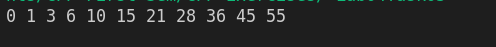Write a c++ program that prints Sum of the series 1, 3, 6, 10… (Triangular Numbers).

Note. Create two separate programs (one using a for loop and the other using a while loop).cpp programming exercise with solution

By using for loop

#include <iostream>
using namespace std;

int main()
{

for (int i = 0; i<= 10; i++)
{
cout<<i*(i+1)/2<<" ";
}

return 0;
}

By using while loop

#include <iostream>
using namespace std;

int main()
{
int i = 0;

while (i<=10)
{
cout<<i*(i+1)/2<<" ";
i++;
}

return 0;
}